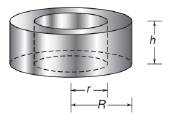Chapter 9.3, Problem 34EElementary Geometry For College St...

7th Edition
Alexander + 2 others
ISBN: 9781337614085

Solutions

Chapter
SectionElementary Geometry For College St...

7th Edition
Alexander + 2 others
ISBN: 9781337614085
Textbook Problem

In Exercises 34 and 35, give a paragraph proof for each claim.The total area T of a right circular cylinder whose altitude is of length h and whose circular bas has a radius of length r is given by T = 2 π r ( r + h ) .To determine

To give:

The paragraph proof for the claim “The total area T of a right circular cylinder whose altitude is of length h and whose circular base has a radius of length r is given by T=2πr(r+h)”.

Explanation

Given:

The claim is “The total area T of a right circular cylinder whose altitude is of length h and whose circular base has a radius of length r is given by T=2πr(r+h)”.

Proof:

Consider a right circular cylinder.

Let r be the radius of the base of the cylinder and h be the altitude of the cylinder.

The lateral area of the right circular cylinder is L=2πrh.

The base of the cylinder is a circle with radius r.

The base area of the right circular cylinder is B=πr2.

Hence the total area of the right circular cylinder is T=L+B

Still sussing out bartleby?

Check out a sample textbook solution.

See a sample solution

The Solution to Your Study Problems

Bartleby provides explanations to thousands of textbook problems written by our experts, many with advanced degrees!

Get Started

What is the slope of a nonvertical line? What can you say about the slope of a vertical line?

Applied Calculus for the Managerial, Life, and Social Sciences: A Brief Approach

In Exercises 15-18, find the limit (if it exists). limx5x+5x5

Calculus: An Applied Approach (MindTap Course List)

In Exercises 4756, solve the given equation for the indicated variable. 423x=256

Finite Mathematics and Applied Calculus (MindTap Course List)